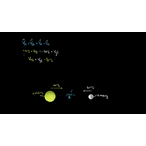# Class 11 Physics (India): How to use the shortcut for solving elastic collisions

Need more information? Get more details on the site of the provider.### Description

In this video, David solves an example elastic collision problem to find the final velocities using the easier/shortcut approach.

## Class 11 Physics (India)

Let's learn, practice, and master topics of class 11 physics (NCERT) starting with kinematics and then moving to dynamics with Newton's laws of motion, work, energy, and power. Let's then use these as the foundation to learn about centre of mass, rotational motion, gravitation, solids, fluids, thermodynamics, and oscillations and waves.

## Elastic and inelastic collisions

Categorizing the different types of collisions can be useful. Here, you'll learn what it means for a collision to be elastic or inelastic and what that implies mathematicall…

There are no frequently asked questions yet. If you have any more questions or need help, contact our customer service.

Didn't find what you were looking for? See also: Physics, Teaching Skills, Biology, Mathematics, and Science.

In this video, David solves an example elastic collision problem to find the final velocities using the easier/shortcut approach.

## Class 11 Physics (India)

Let's learn, practice, and master topics of class 11 physics (NCERT) starting with kinematics and then moving to dynamics with Newton's laws of motion, work, energy, and power. Let's then use these as the foundation to learn about centre of mass, rotational motion, gravitation, solids, fluids, thermodynamics, and oscillations and waves.

## Elastic and inelastic collisions

Categorizing the different types of collisions can be useful. Here, you'll learn what it means for a collision to be elastic or inelastic and what that implies mathematically.

## Topic: Science

Learn about all the sciences, from physics, chemistry and biology, to cosmology and astronomy, across hundreds of videos, articles and practice questions.

There are no reviews yet.
• View related products with reviews: Physics.